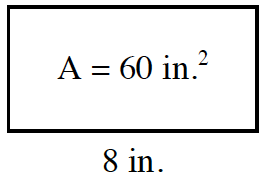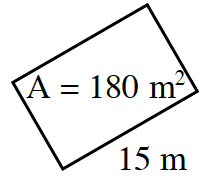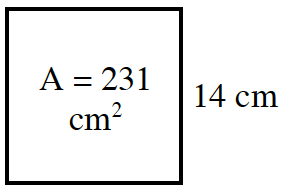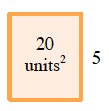### Home > CC3 > Chapter 1 > Lesson 1.1.4 > Problem1-35

1-35.

The area of each rectangle below is shown in the middle of the rectangle. For each figure, find the missing length or width. Homework Help ✎

1.The area of a rectangle is the product of the length and width.

Substitute the area and the unknown
length or the width into the equation
$A=l·w$.

Solve for the unknown variable.

$60=$ length $·\ 8$

$\frac{ 60 }{ 8} = \frac{ \text{length }\cdot 8 }{8 }$

$\text{length }= \frac{ 60 }{8 } = 7.5$

1.Try not to be confused by the orientation of the rectangle. Solve as in part (a).

1.See the example below.$20=5\ ·$ length

$\frac{ 20 }{ 5} = \frac{ 5 \cdot \text{length} }{5 }$

$\text{length }= \frac{ 20 }{ 5} = 4$